# Pyramids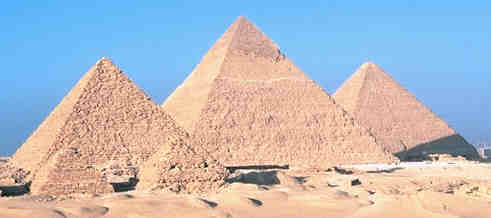When we think of pyramids we think of the Great Pyramids of Egypt.

They are actually Square Pyramids, because their base is a Square.

## Parts of a PyramidA pyramid is made by connecting a base to an apex

The base is a polygon (flat with straight edges) and all other faces are triangles. No curves!

## Types of Pyramids

There are many types of Pyramids, and they are named after the shape of their base.

Triangular Square Pentagonal Pyramid: Pyramid: Pyramid: Pyramid Base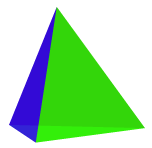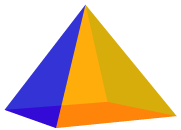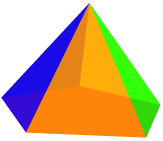Fly through some pyramids here.

## Right vs Oblique Pyramid

This tells us where the top (apex) of the pyramid is. When the apex is directly above the center of the base it is a Right Pyramid, otherwise it is an Oblique Pyramid.

Right Pyramid Oblique Pyramid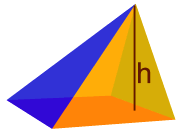## Regular vs Irregular Pyramid

This tells us about the shape of the base. When the base is a regular polygon it is a Regular Pyramid, otherwise it is an Irregular Pyramid.

Regular Pyramid Irregular Pyramid## Area and Volume### The Volume of a Pyramid

• 1/3 × [Base Area] × Height

### The Surface Area of a Pyramid

When all side faces are the same:

• [Base Area] + 1/2 × Perimeter × [Slant Length]

When side faces are different:

• [Base Area] + [Lateral Area]

### Notes On Surface Area

The Surface Area has two parts: the area of the base (the Base Area), and the area of the side faces (the Lateral Area).

For Base Area :

It depends on the shape, there are different formulas for triangle, square, etc. See Area for formulas, or our Area Calculation Tool

For Lateral Area :

When all the side faces are the same:

• Multiply the perimeter by the "slant length" and divide by 2. This is because the side faces are always triangles and the triangle formula is "base times height divided by 2"

But when the side faces are different (such as an "irregular" pyramid) we must add up the area of each triangle to find the total lateral area.